## Feasible and Realizable Paths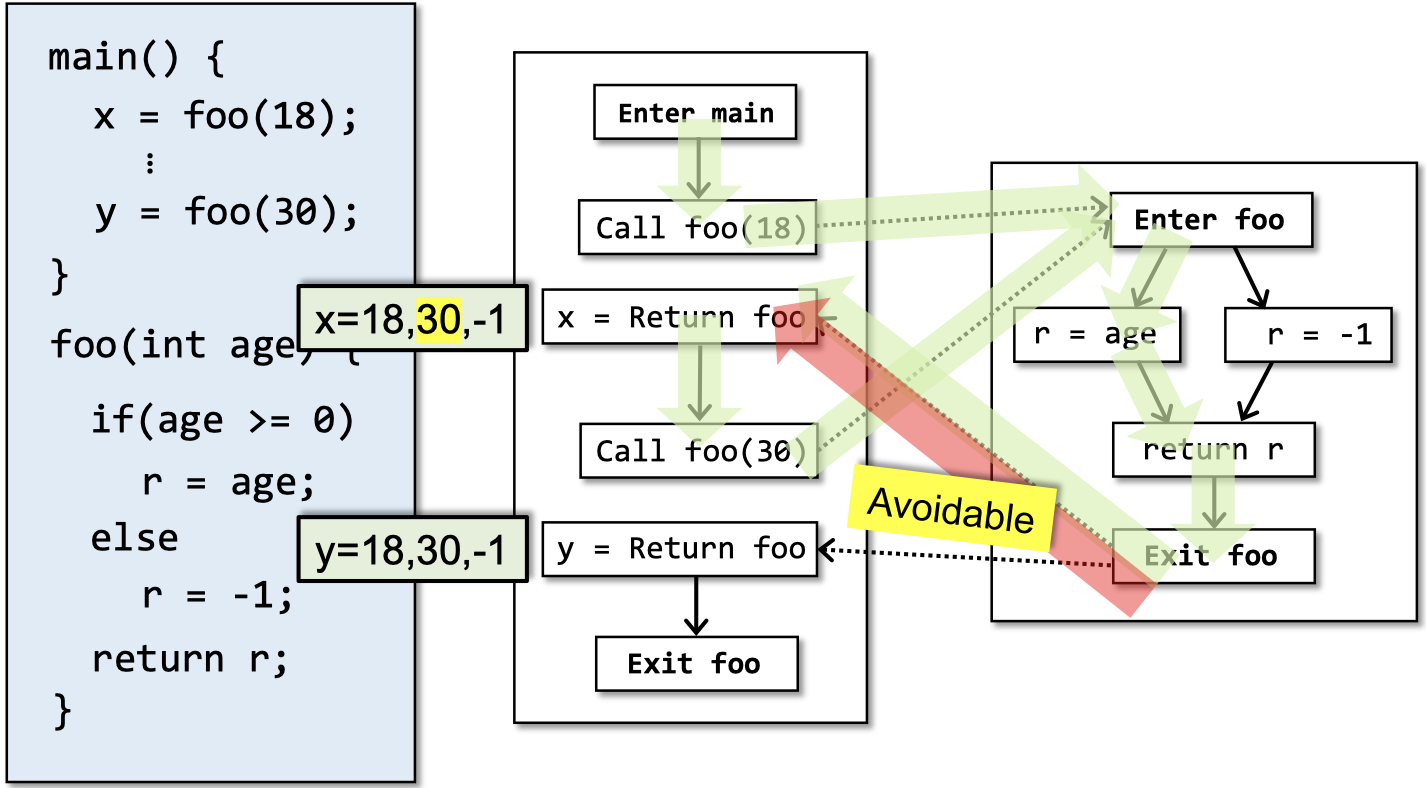## CFL-Reachability

CFL即context-free language，上下文无关语言。一个路径连接了CFG上的节点A和B，或者说B是从A可达的，当且仅当从A到B的路径的边的标签连接起来构成的字符串符合上下文无关语言文法规则。

$$S \rightarrow \alpha$$

$$S \rightarrow aSb \\ S \rightarrow \varepsilon$$

• 在每个右括号$)_{i}$之前，都必须有一个对应的左括号$(_{i}$匹配。但是反过来则不必满足——每个左括号$(_{i}$后面不一定要跟着一个右括号$)_{i}$。
• 对于每个call site $i$来说，我们将其call edge和return edge分别标记为$(_{i}$和$)_{i}$。
• 将CFG中剩下的边标记为符号$e$。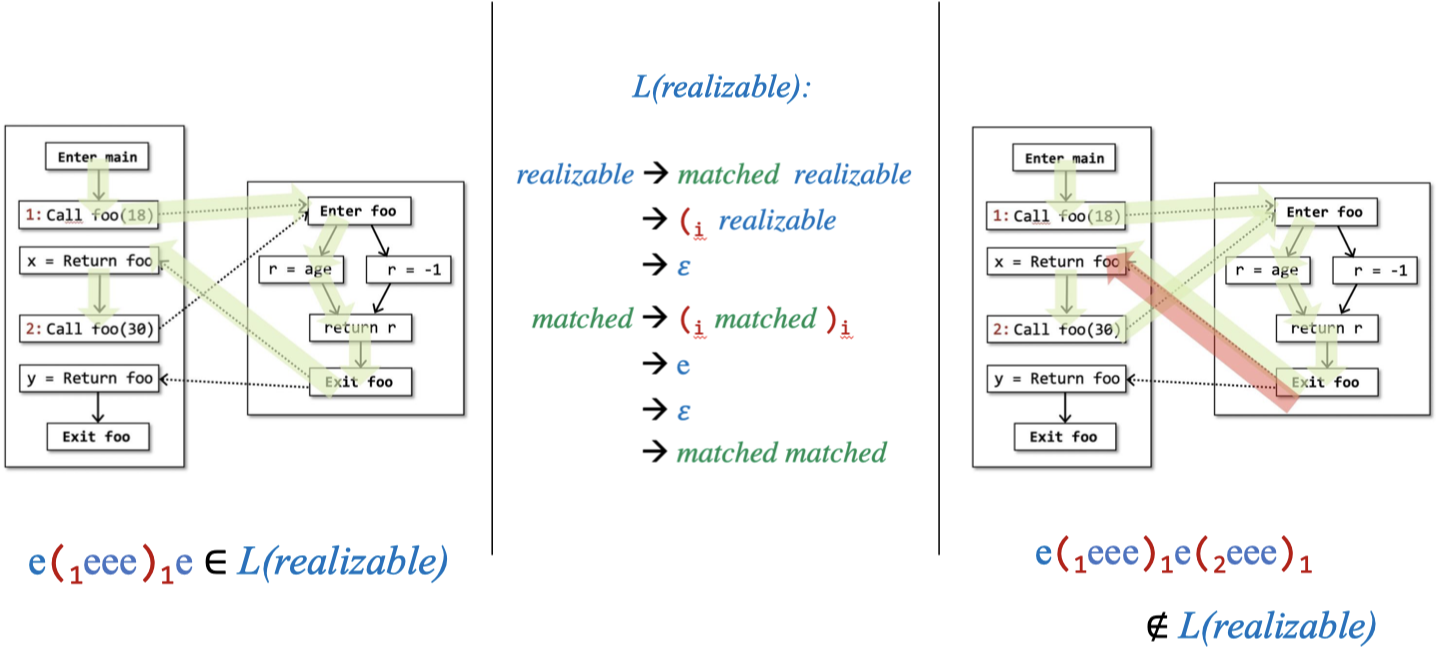## IFDS简介

MRP是什么呢？我们在《南大软分课程笔记｜06 数据流分析理论》中学习过MOP，这里简单回顾一下（之前应用在节点上，现在我们应用在边上，思想是一样的）：

$$pf_{p} = f_{n} \circ\ …\ \circ f_{2} \circ f_{1}$$

$$\text{MOP}_{n} = \bigsqcup_{p\ \in\ \text{Paths}(start, n)} pf_{p}(\bot)$$

$$\text{MRP}_{n} = \bigsqcup_{p\ \in\ \text{RPaths}(start, n)} pf_{p}(\bot)$$

• 为$P$构建一个supergraph $G^{\star}$，并基于$Q$为$G^{*}$中的每个边定义flow function。
• 为$P$构建一个exploded supergraph $G^{\sharp}$，构建方式是将flow functions转化为表示关系（representation relations）图。
• 问题$Q$可以通过在$G^{\sharp}$上应用Tabulation算法，作为图可达性问题被解决。其实质就是找出所有的MRP解。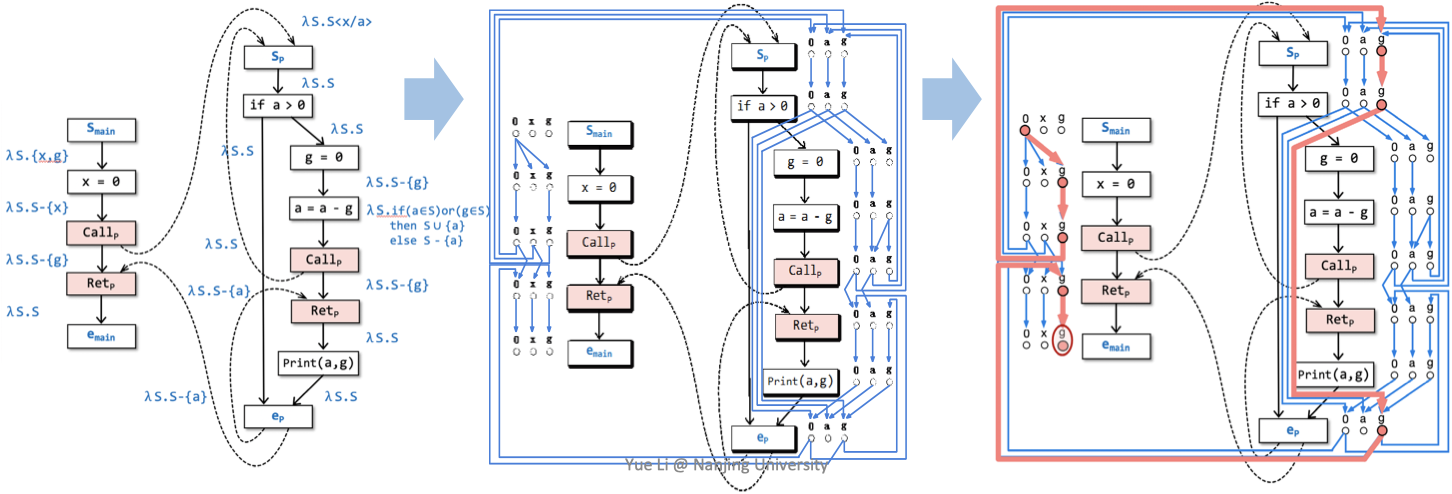## Supergraph和Flow Functions

$G^{\star}$中的每个过程调用包含三条边：

• 一个从$Call_{p}$到$Ret_{p}$的过程间call-to-return边
• 一个从$Call_{p}$到被调用过程的$s_{p}$的过程间call-to-start边
• 一个从被调用过程的$e_{p}$到$Ret_{p}$的exit-to-return-site边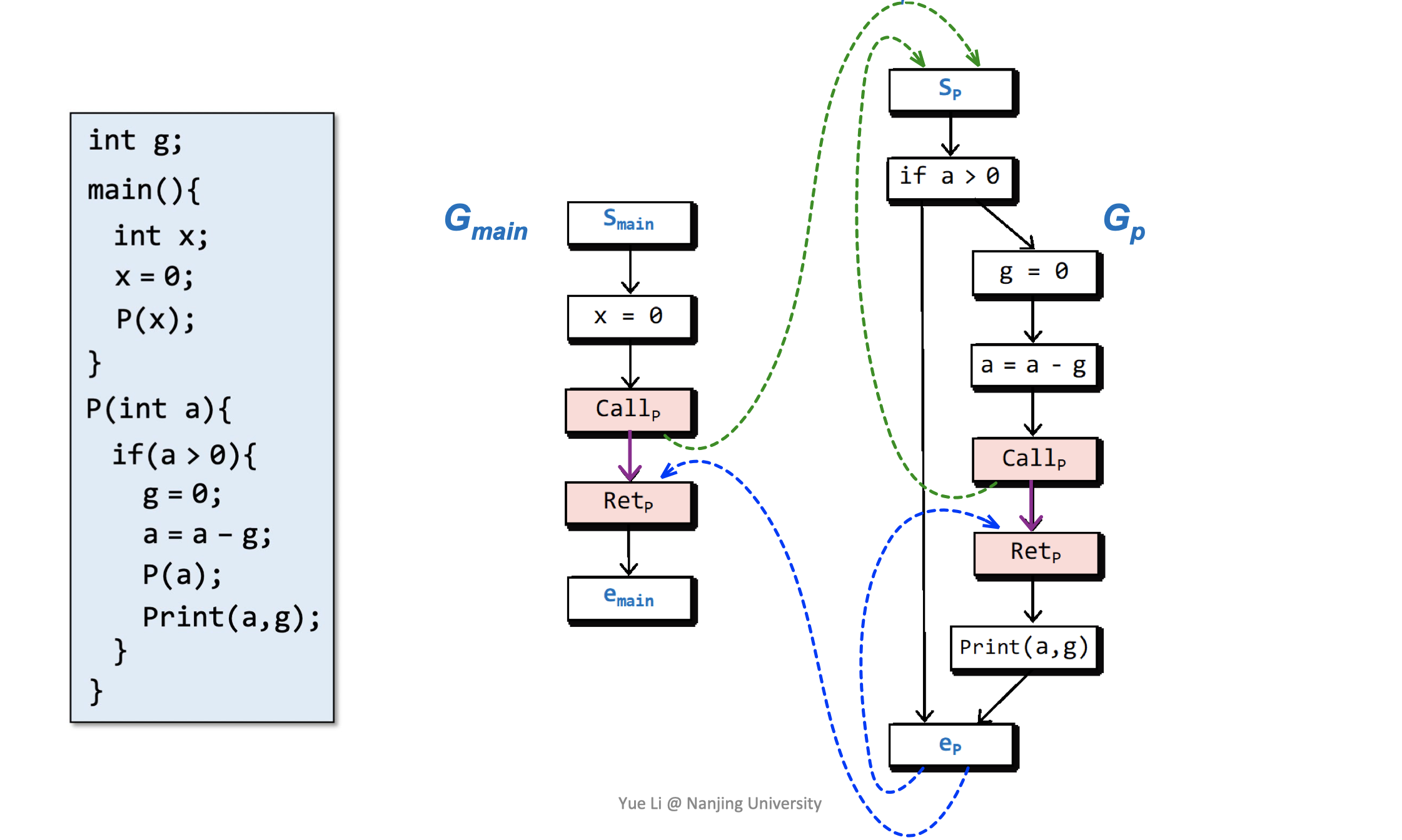“可能的未初始化变量”分析指的是，对于每个节点$n \in N^{\star}$，确定在执行流到达$n$之前未初始化的变量集合。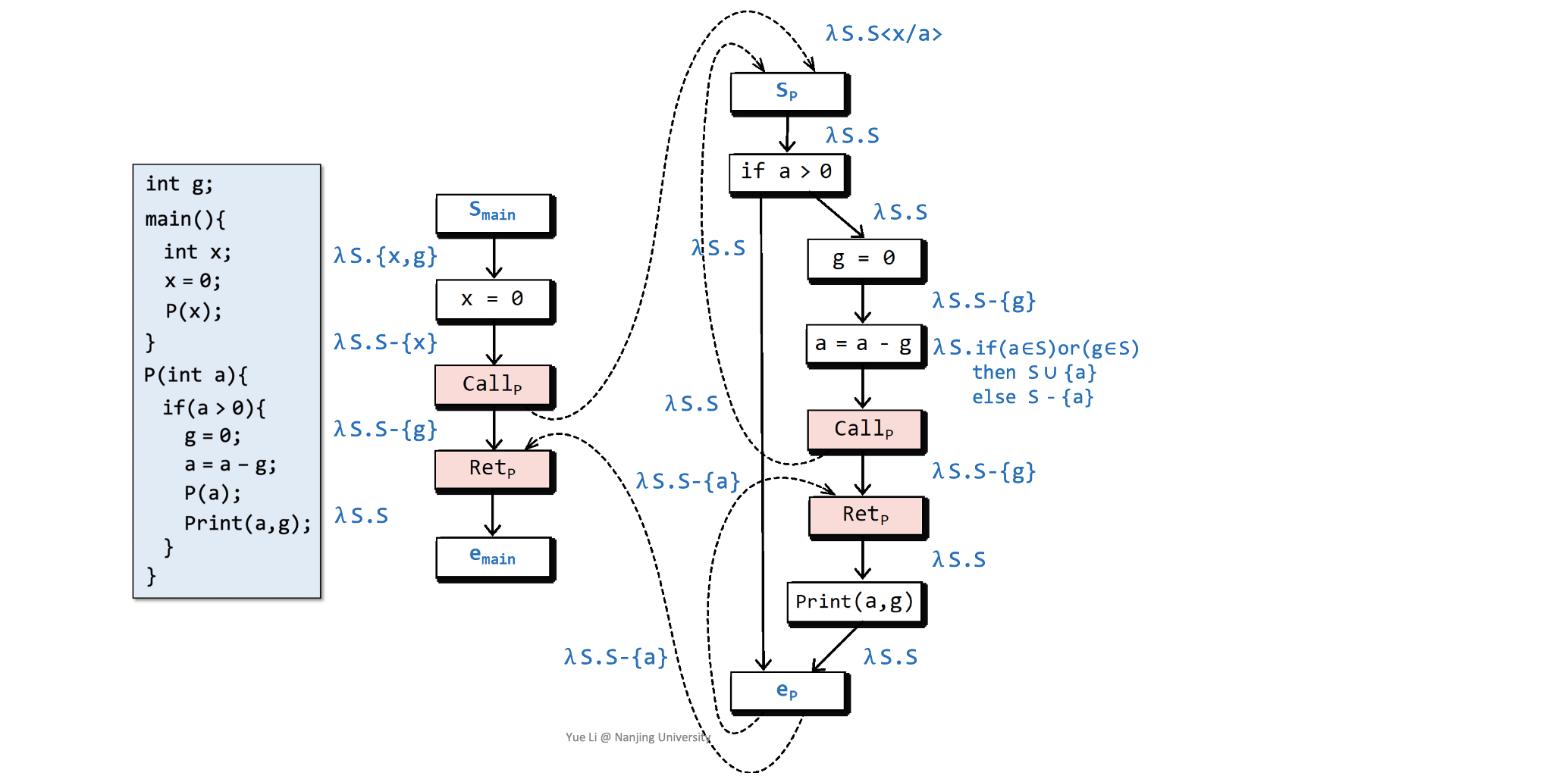## Exploded Supergraph和Tabulation算法

OK，现在有了supergraph和flow functions，我们接下来构建exploded supergraph $G^{\sharp}$，构建方式是将flow functions转化为representation relations。每个flow function可以用一个图来表示，图中有$2(D+1)$个节点，最多有$(D+1)^{2}$条边，$D$是data facts的有限集。

$$R_{f} = \lbrace (0, 0) \rbrace \ \cup \ \lbrace (0, y) \ | \ y \in f(\empty) \rbrace \ \cup \ \lbrace (x, y) \ | \ y \notin f(\empty) \ \text{and} \ y \in f(\lbrace x \rbrace) \rbrace$$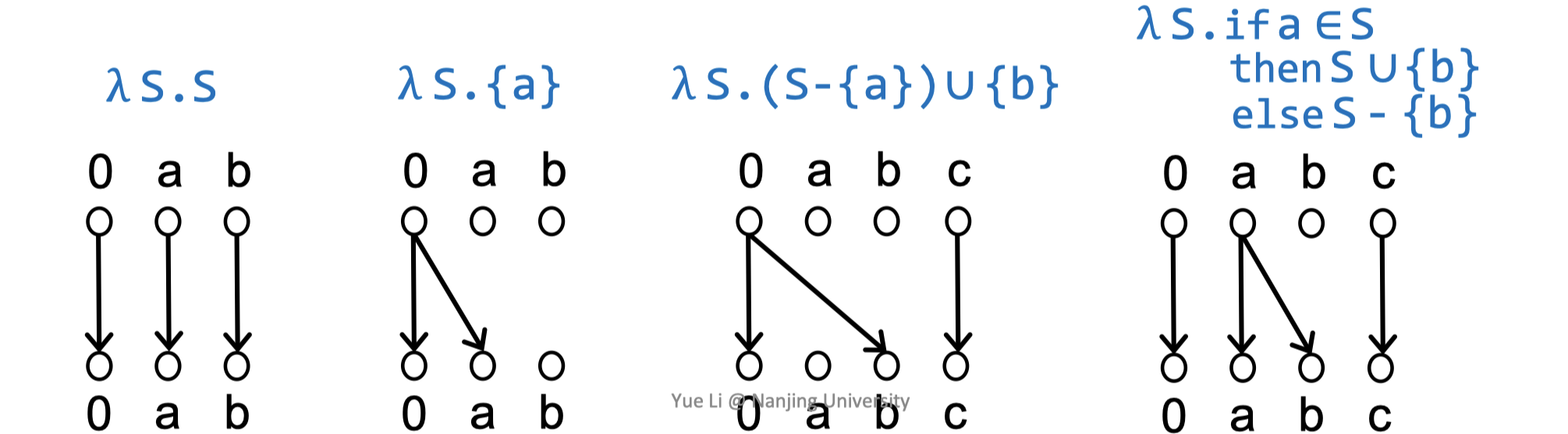Exploded有“爆裂的”之意。Exploded supergraph $G^{\sharp}$指的是，$G^{\star}$中的每个节点$n$都“爆裂”为$G^{\sharp}$中的$D+1$个节点，$G^{\star}$中的每个$n_{1} \rightarrow n_{2}$的边也“爆裂”为$G^{\sharp}$中的representation relation（借助flow funciton转化）。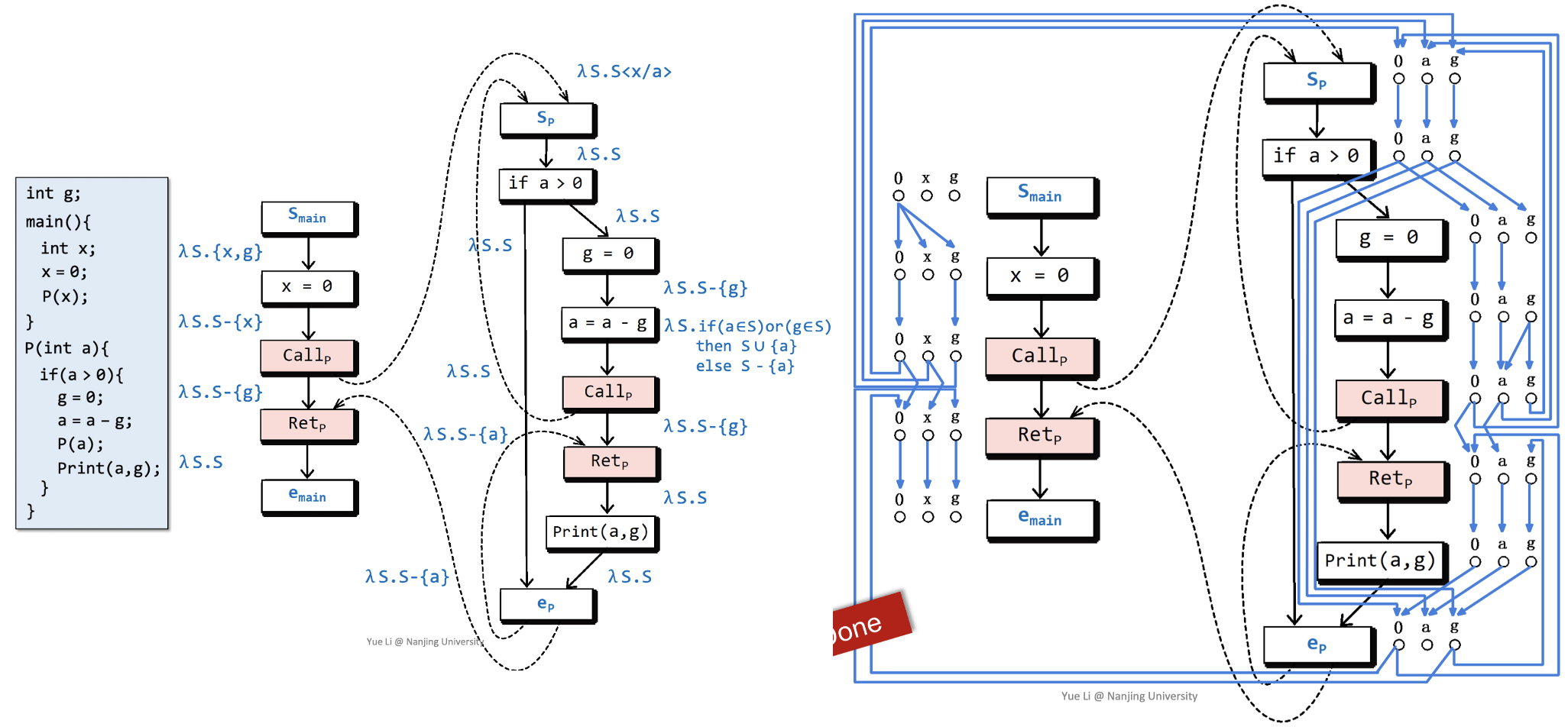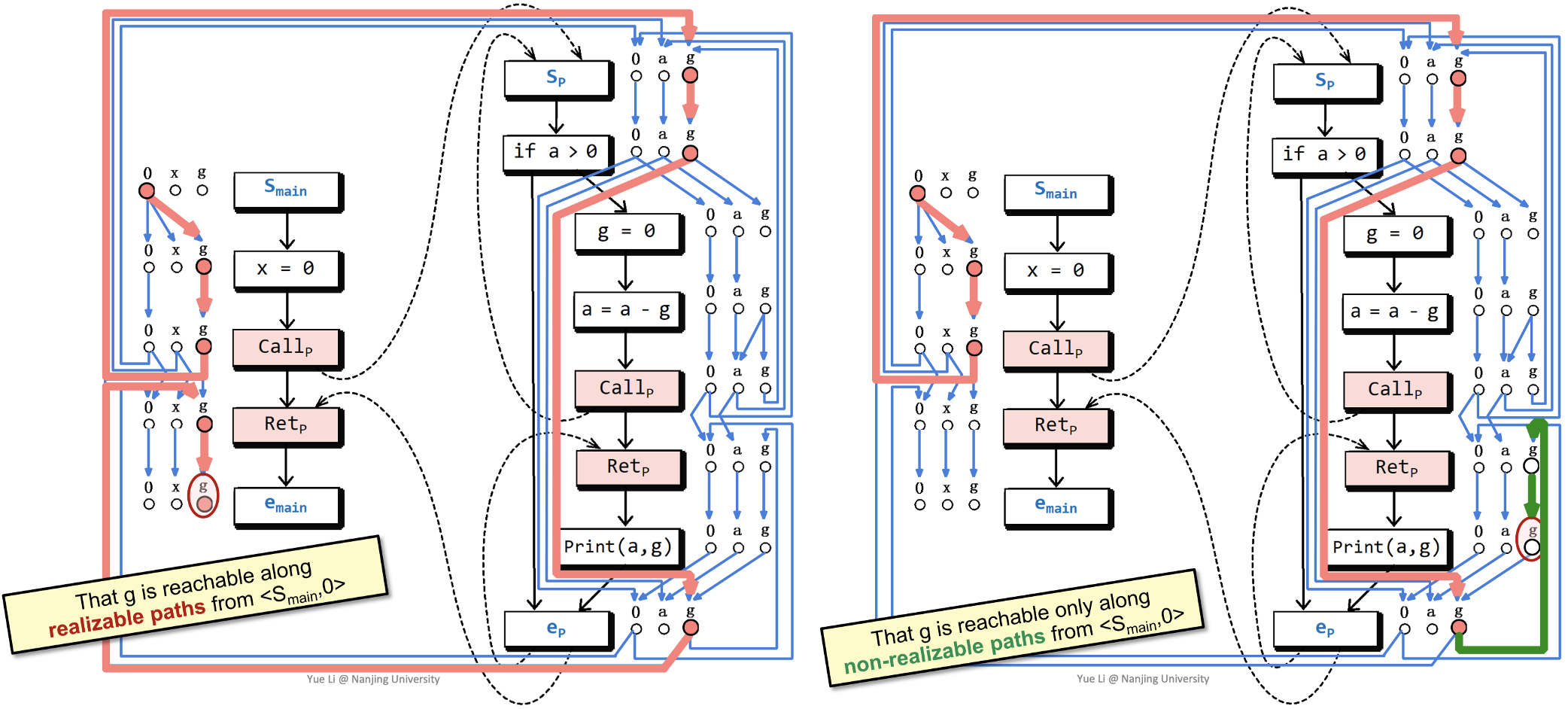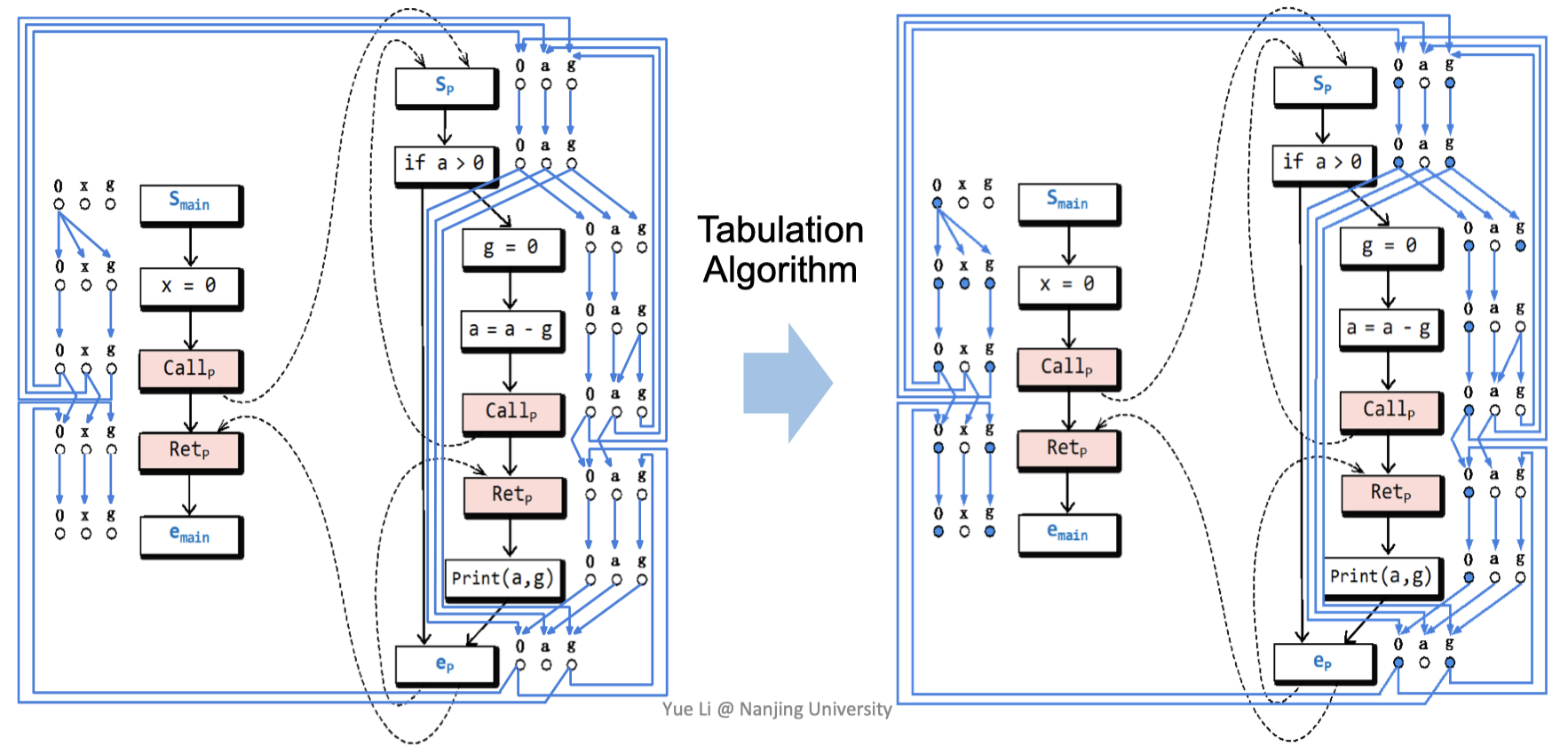Tabulation算法是一种动态规划技术，用于通过将问题分解成较小的、重叠的子问题，并将结果存储在表格中以实现高效重用，从而解决问题。这种方法对于优化和组合问题特别有效，因为它有助于避免冗余计算并加速解决过程。

Tabulation算法的基本思想是构建一个表示问题解空间的表格（通常是一个二维数组）。然后，算法通过迭代地填充表格，使每个条目对应于特定子问题的解决方案。通常通过分析表格中的值来找到整个问题的最终解决方案。

Tabulation算法与另一种动态规划技术（称为Memoization）的区别在于，Tabulation算法采用自底向上的方法，先解决较小的子问题，然后构建整个问题，而Memoization采用自顶向下的方法，通过将整个问题分解成较小的子问题并缓存其结果来解决问题。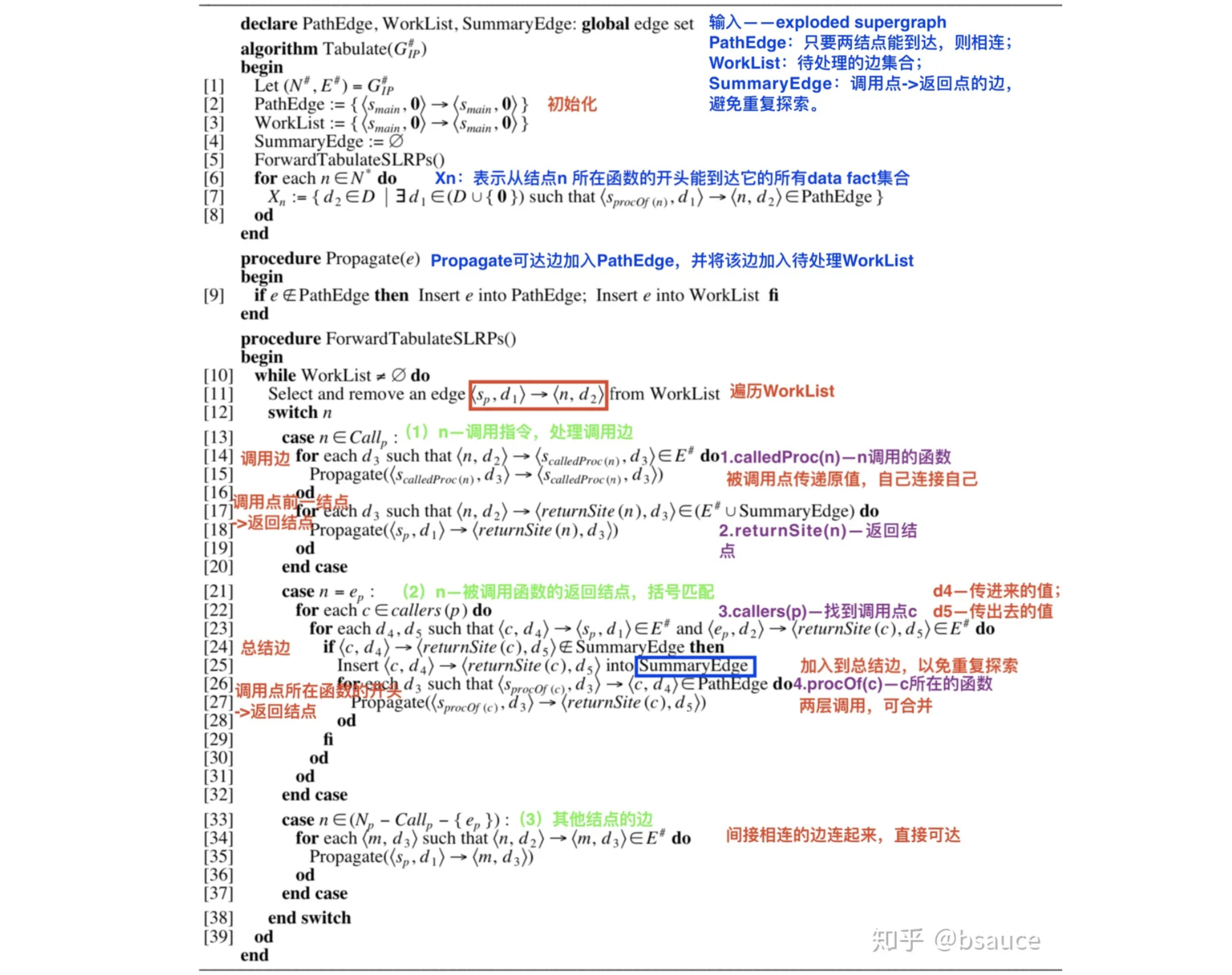## IFDS的可分配性（Distributivity）

IFDS中的I、F、S都比较好理解，那么D对应的“可分配”又如何理解呢？

$$F(x \wedge y) = F(x) \wedge F(y)$$

IFDS中的每个flow function每次处理的输入data fact都只有一个，而常量传播中的一次处理可能会依赖多于一个data fact的情况，例如$z = x + y$，我们需要同时给出$x$和$y$的情况作为输入。在IFDS中，我们没法定义一个同时依赖$x$和$y$的$F$。因此，类似的分析都不能用IFDS来做。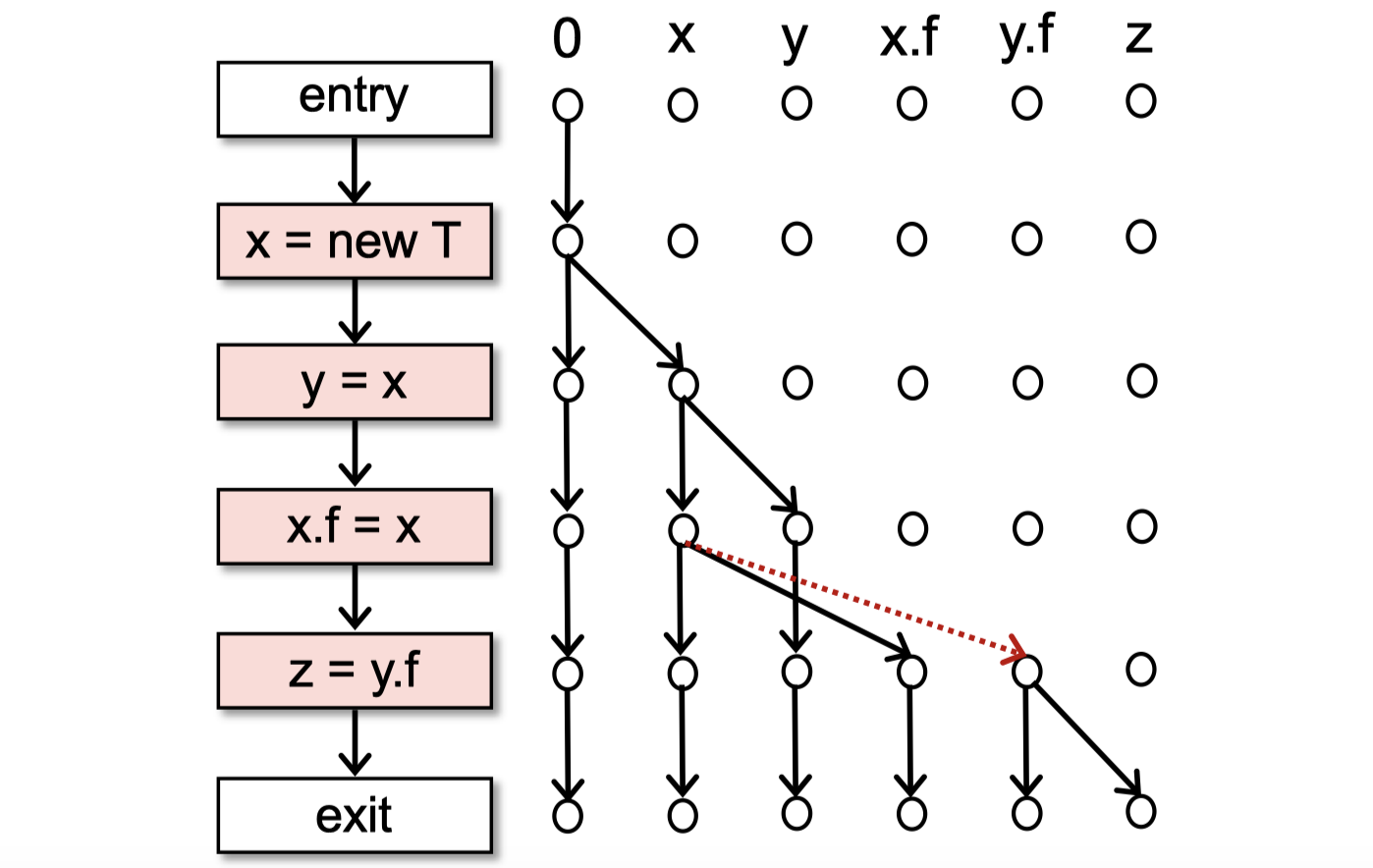## Soundiness

• 一个所谓的sound的静态分析，只是在实现上有一个sound core，也就是对绝大多数语言特性做了over-approximated处理，但是某些难以分析的部分则是under-approximated处理。
• 略去hard language features的处理，或者只是在实现或评估部分简单地提一下。
• 未能考虑到hard language features，导致分析结果有问题。

1. 字符串常量分析+指针分析（论文链接）。这个思路比较直观，但是也存在明显的问题——如果字符串的值确实是运行时才能确定的，那么就无法确定反射目标了。
2. 类型推理+字符串分析+指针分析（论文链接，一二作是李樾、谭添）。这个思路是从反射对象的使用点（usage points）开始反推。值得一提的是，2019年，两位老师又发表了新的研究成果
3. 动态分析辅助的静态分析（论文链接）。这个不必多言，就是从动态分析中获取信息来辅助进行静态分析，它会有动态分析的优点，但是也因此引入了动态分析的缺点。

Java native code难于分析的原因则是跨语言——最终会调用到C语言动态链接库中的代码。当前的一个解决思路是对关键的native code进行手动建模（用Java实现）。这里附上2020年的一个研究进展